# Workflow example for the steppe bison

#### 2021-10-14

In this vignette, we demonstrate the new features that paleopop adds to poems for modeling populations over paleo-ecological time scales using the example of the steppe bison in Siberia. The steppe bison was once distributed throughout the Northern Hemisphere. It became regionally extinct in Siberia 9,500 years ago, and it is thought to have gone completely extinct in Canada 500 years ago. We will model the range dynamics of the steppe bison in Siberia from the Last Glacial Maximum (21,000 years ago) until 9,000 years before present. Along the way, we will learn how paleopop handles regions, model templates, simulations, and results.

# Setup

Here we load poems and paleopop and set the number of parallel cores for simulation and an output directory for results.

library(poems)
library(paleopop)
parallel_cores <- 2
output_dir <- tempdir()

In this vignette, we will do the following:

1. Create a PaleoRegion

2. Generate dispersal rates and carrying capacities

3. Create the model template

4. Sample parameter space with Latin Hypercube Sampling

5. Run the simulations

6. Examine the results

# 1. Create a PaleoRegion

While poems implements a static region object, in paleopop we use something different, because regions on paleo time scales are never static. Oceans rise, continents collide, and the contours of our spatially explicit landscape shift. In the case of the steppe bison in Siberia, the sea level rises over time. paleopop includes a temporally dynamic raster stack of Siberia we can use to build a PaleoRegion object. The raster resolution is 2 by 2.

library(raster)
raster::plot(paleopop::siberia_raster[], main = "Siberia raster (LGM)",
xlab = "Longitude (degrees)", ylab = "Latitude (degrees)",
colNA = "blue")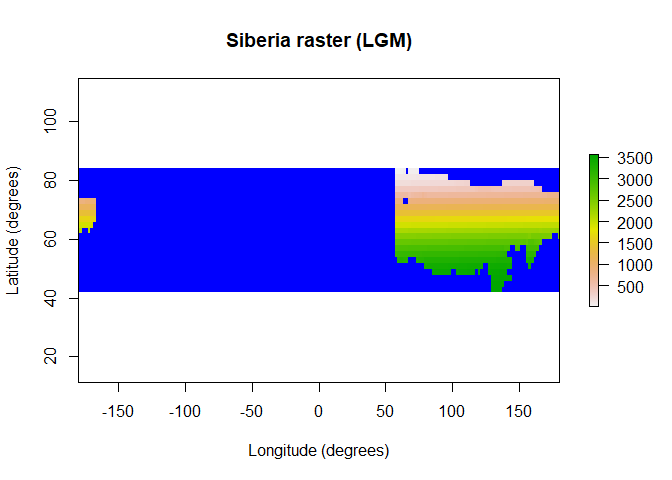raster::plot(paleopop::siberia_raster[], main = "Siberia raster (9000 BP)",
xlab = "Longitude (degrees)", ylab = "Latitude (degrees)",
colNA = "blue")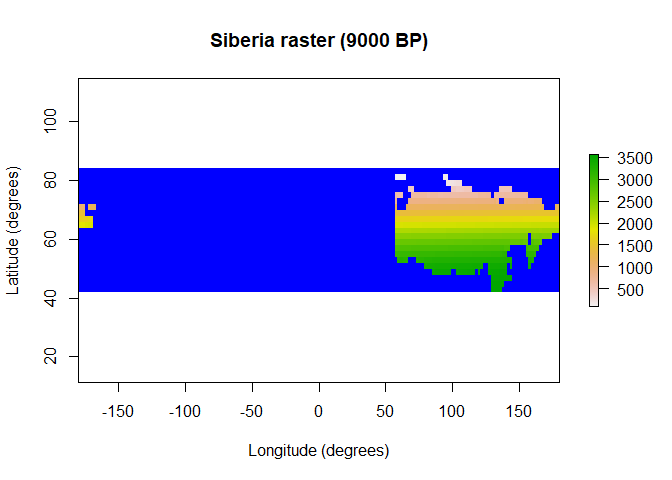We can use this RasterStack object as a template to create a PaleoRegion object, which will set the indices of occupiable cells at each time step, and mask cells that are not occupiable at a time step (in the case of this example, because those cells are now underwater.) When we plot the region_raster in the PaleoRegion object, we see the maximum extent of the region, showing all cells that are occupiable at any time step. The temporal_mask_raster method generates a RasterStack that marks occupiable cells at each time step with a 1.

region <- PaleoRegion$new(template_raster = paleopop::siberia_raster) raster::plot(region$region_raster, main = "Bison region maximum extent (cell indices)",
xlab = "Longitude (degrees)", ylab = "Latitude (degrees)",
colNA = "blue")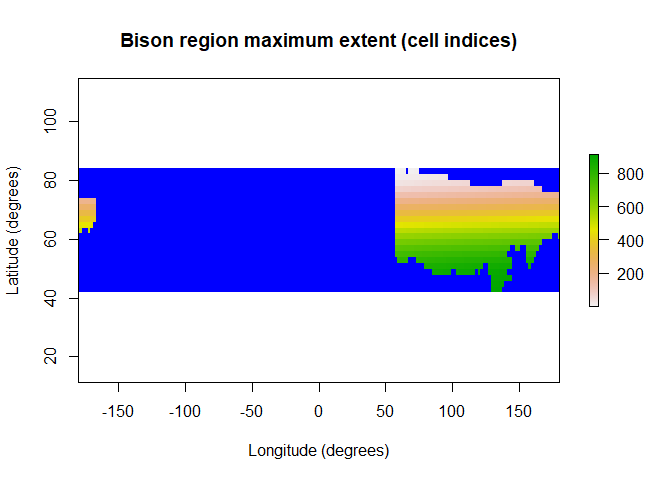# 2. Generate dispersal and carrying capacity

This part of the workflow is identical to poems: we create Generator objects to dynamically generate dispersal between grid cells, initial abundance, and carrying capacity at each point in space and time.

## 2.a. Generate dispersal matrix

We cut down on computational time during the simulations by generating ahead of time a matrix that describes the potential dispersal rates between every pairwise combination of cells in the study region. Dispersal rates are calculated using a distance-based dispersal function as well as, optionally, a conductance/friction landscape that maps out barriers to dispersal, such as ice or mountains. (We will omit the friction landscape for this example.)

dispersal_gen <- DispersalGenerator$new(region = region, dispersal_max_distance = 500, # km distance_classes = seq(10, 500, 10), # divide into bins distance_scale = 1000, # sets units to km dispersal_friction = DispersalFriction$new(), # empty
inputs = c("dispersal_p", "dispersal_b"),
decimals = 3)
dispersal_gen$calculate_distance_data(use_longlat = TRUE) # pre-calculate matrix of distances between cells test_dispersal <- dispersal_gen$generate(input_values =
list(dispersal_p = 0.5,
dispersal_b = 200))$dispersal_data head(test_dispersal[]) #> target_pop source_pop emigrant_row immigrant_row dispersal_rate #> 1 2 1 1 1 0.076 #> 2 3 1 2 1 0.065 #> 3 4 1 3 1 0.056 #> 4 9 1 4 1 0.028 #> 5 10 1 5 1 0.028 #> 6 11 1 6 1 0.026 ## 2.b. Generate carrying capacity landscape Here we will generate a dynamic landscape based on a raster stack of habitat suitability values (from 0, totally unsuitable, to 1, ideally suitable). paleopop has a spatiotemporally explicit habitat suitability RasterStack for Siberia from 21,000 - 9,000 years BP. raster::plot(bison_hs_raster[], main = "Bison habitat suitability (LGM)", xlab = "Longitude (degrees)", ylab = "Latitude (degrees)", colNA = "blue")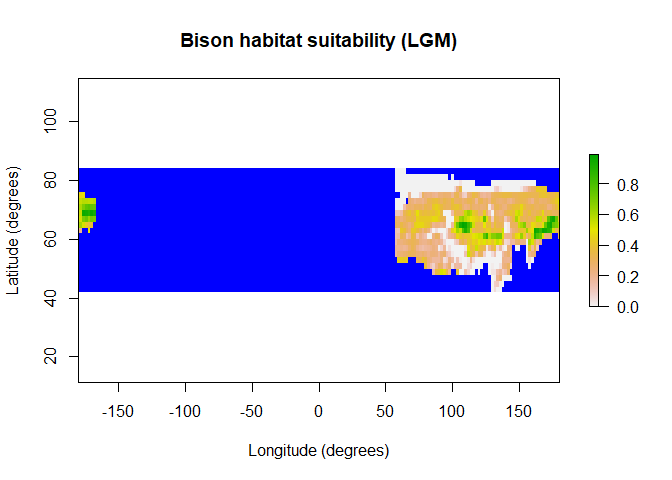However, PaleoPop simulations are not based on habitat suitability values. Rather, they operate on initial populations and on carrying capacities. Therefore, we need to enter these habitat suitability landscapes into a generator that can translate them into an initial abundance landscape and into a dynamic carrying capacity landscape. To do this, we must create a poems::Generator object. Because every simulation has different requirements, we must set our own inputs, outputs, and generator functions to convert the inputs into the outputs. Here, we will very simply use a maximum density parameter and multiply it by the habitat suitability. # Convert the habitat suitability raster to matrix bison_hs <- bison_hs_raster[region$region_indices]
# Create the generator
capacity_gen <- Generator$new(description = "Capacity generator", example_hs = bison_hs, inputs = c("density_max"), outputs = c("initial_abundance", "carrying_capacity")) # indicate that initial abundance and carrying capacity are required capacity_gen$add_generative_requirements(list(initial_abundance = "function",
carrying_capacity = "function"))
# define custom generative functions
capacity_gen$add_function_template("carrying_capacity", function_def = function(params) { round(params$density_max*params$example_hs) }, call_params = c("density_max", "example_hs")) capacity_gen$add_function_template("initial_abundance",
function_def = function(params) {
params$carrying_capacity[,1] }, call_params = c("carrying_capacity")) # test run test_capacity <- capacity_gen$generate(input_values = list(density_max = 2000))
raster::plot(region$raster_from_values(test_capacity$initial_abundance), main = "Initial abundance",
xlab = "Longitude (degrees)", ylab = "Latitude (degrees)",
colNA = "blue")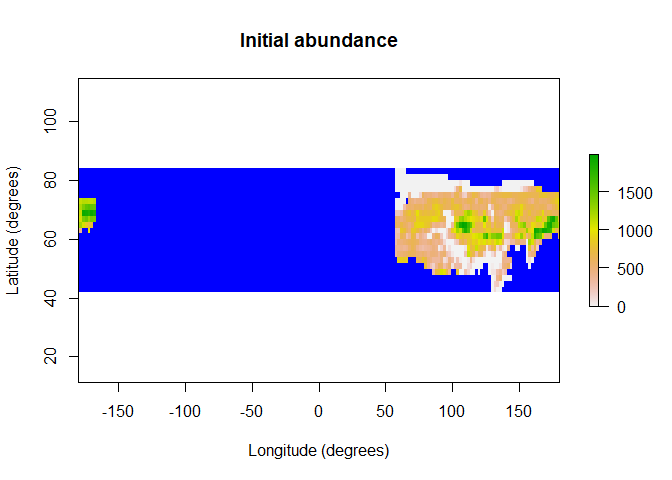# 3. Create the model template

We create a model template for simulation using the PaleoPopModel object. Let’s check the required attributes for the object.

model_template <- PaleoPopModel$new() model_template$model_attributes # all possible attributes
#>   "coordinates"           "random_seed"           "time_steps"
#>   "years_per_step"        "populations"           "initial_abundance"
#>   "transition_rate"       "standard_deviation"    "compact_decomposition"
#>  "carrying_capacity"     "density_dependence"    "growth_rate_max"
#>  "dispersal_data"        "dispersal_target_k"    "harvest"
#>  "harvest_max"           "harvest_g"             "harvest_z"
#>  "harvest_max_n"         "human_density"         "abundance_threshold"
#>  "occupancy_threshold"   "results_selection"
model_template$required_attributes # required attributes to run simulations #>  "time_steps" "years_per_step" "populations" #>  "initial_abundance" "transition_rate" "standard_deviation" #>  "carrying_capacity" "harvest" "results_selection" Initial abundance, carrying capacity, and dispersal data will be provided by the generators above. Here we will define an environmental correlation matrix so there can be spatial autocorrelation in the simulations. # Distance-based environmental correlation (via a compacted Cholesky decomposition) env_corr <- SpatialCorrelation$new(region = region, amplitude = 0.4, breadth = 500)
correlation <- env_corr$get_compact_decomposition(decimals = 2) Normally we would have spatiotemporally dynamic data on human density over time, which the prey- and predator-dependent harvest function translates into numbers of bison harvested. The harvest function in paleopop is defined by harvest_z, which determines whether harvesting is a Type II or Type III functional response, harvest_g, a constant added to the denominator that reduces harvest rates, and harvest_max_n, a ceiling on maximum prey density that can be harvested. In the interest of simplicity for this example, we will create a matrix of constant low human density. human_density <- array(rep(0.1), c(913, 1001)) # rows = populations and columns = timesteps Now we have all the components we need to build the model template, less the model attributes that will be sampled via Latin Hypercube Sampling later. model_template <- PaleoPopModel$new(
simulation_function = "paleopop_simulator", # this is the default; made it explicit for the example
region = region,
# coordinates: you could use XY coordinates to define the region instead
time_steps = 1001,
years_per_step = 12, # generational length for bison
populations = region$region_cells, # extracts number of population cells # initial_abundance: generated transition_rate = 1.0, # rate of transition between generations # standard_deviation: sampled compact_decomposition = correlation, # carrying_capacity: generated density_dependence = "logistic", # growth_rate_max: sampled harvest = TRUE, # harvest_max: sampled harvest_g = 0.4, # constant # harvest_z: sampled # harvest_max_n: sampled human_density = human_density, dispersal_target_k = 10, # minimum carrying capacity that attracts dispersers # dispersal_data: generated # abundance_threshold: sampled # initial_n: sampled occupancy_threshold = 1, # threshold: # of populations for the species to persist random_seed = 321, results_selection = c( # ema (expected minimum abundance), extirpation, occupancy, human_density, "abundance", "harvested" ) ) # 4. Sample model and generator parameters for each simulation Here the workflow is the same as in poems: we sample along a range of values so as to create a prior, and use these to parameterize different simulations. Here, we will sample each range of values uniformly so as to create an uninformative prior, but the LHS generator object offers options for sampling along different distributions to create an informative prior. For the sake of this example, we will sample 100 different parameter combinations, but it is advisable to run more in order to fully sample the parameter space. nsims <- 100 # adjust to run your own example if desired lhs_generator <- LatinHypercubeSampler$new()
lhs_generator$set_uniform_parameter("standard_deviation", lower = 0, upper = sqrt(0.06)) lhs_generator$set_uniform_parameter("growth_rate_max", lower = log(1.31), upper = log(2.84))
lhs_generator$set_uniform_parameter("abundance_threshold", lower = 0, upper = 500, decimals = 0) lhs_generator$set_uniform_parameter("harvest_max", lower = 0, upper = 0.35)
lhs_generator$set_uniform_parameter("harvest_z", lower = 1, upper = 2) lhs_generator$set_uniform_parameter("density_max", lower = 500, upper = 3250) # alias for harvest_max_n
lhs_generator$set_uniform_parameter("dispersal_p", lower = 0.05, upper = 0.25) # for the dispersal generator lhs_generator$set_uniform_parameter("dispersal_b", lower = 65, upper = 145) # for the dispersal generator

sample_data <- lhs_generator$generate_samples(number = nsims, random_seed = 123) sample_data$sample <- c(1:nsims)
#>   standard_deviation growth_rate_max abundance_threshold harvest_max harvest_z
#> 1         0.17996568       0.5978443                 356 0.024664478  1.772628
#> 2         0.08511681       0.3680464                 340 0.005932868  1.193633
#> 3         0.04236395       0.6346779                  73 0.236465865  1.976887
#> 4         0.01406792       0.4795339                 122 0.087687379  1.533396
#> 5         0.12360854       0.6934444                 443 0.096502258  1.917038
#> 6         0.23995795       0.4923823                 423 0.045919751  1.075503
#>   density_max dispersal_p dispersal_b sample
#> 1    2149.138  0.19097699    96.58226      1
#> 2    2641.814  0.07555059    78.71136      2
#> 3    1251.060  0.23121117    78.59283      3
#> 4    3242.684  0.09800821   131.21753      4
#> 5    1150.574  0.08857870   134.67779      5
#> 6    1442.226  0.16579672    88.20734      6

# 5. Run the simulations

Here we build a simulation manager to manage the sample data, generators, and model template. The simulation manager will use the paleopop_simulator function to run simulations.

sim_manager <- SimulationManager$new(sample_data = sample_data, model_template = model_template, generators = list(capacity_gen, dispersal_gen), parallel_cores = parallel_cores, results_dir = output_dir) sim_manager$results_filename_attributes <- c("sample", "results")

run_output <- sim_manager$run() run_output$summary
#>  "100 of 100 sample models ran and saved results successfully"

The simulation log, run_output, can be examined for error messages and failure indices if any of the simulations fail. There is also a detailed simulation log created in the output directory.

# 6. Examine the results

We can explore the results of the simulations using the convenient wrapper of the PaleoPopResults object. Each PaleoPopResults object can hold the result of one simulation, and it can generate outputs that were not specified in the results selection above, such as extirpation times for each population.

results1 <- PaleoPopResults$new( results = readRDS(paste0(output_dir, "/sample_1_results.RData")), region = region, time_steps = 1001 ) head(results1$extirpation)
#>  1 1 1 1 2 2

We can also use a PaleoPopResults object as a kind of template for the ResultsManager, an object that can calculate summary metrics for many results all at once. As with the generators, we must define our own custom metrics functions, because each in silico experiment calls for a different set of metrics to summarize the outputs. We can use the metrics generated by the PaleoPopResults object as the basis for our custom summary metrics.

results_model <- PaleoPopResults$new(region = region, time_steps = 1001, trend_interval = 1:10) metrics_manager <- ResultsManager$new(simulation_manager = sim_manager,
simulation_results = results_model,
generators = NULL) # don't need generators
metrics_manager$summary_metrics <- c("abundance_trend", "extinction_time") metrics_manager$summary_functions <- list()

metrics_manager$summary_functions$extinction_time <- function(simulation_results) {
extinction_time <- -12*(1001 - simulation_results$all$extirpation)-9001 # converts timestep to years BP
if (is.na(extinction_time)) {
extinction_time <- -9001 # if NA, then it survived to the end of the simulation
}
return(extinction_time)
}

metrics_manager$summary_functions$abundance_trend <- function(simulation_results) {
abundance_trend <- simulation_results$all$abundance_trend
return(abundance_trend) # this will use the trend interval specified above
}

gen_log <- metrics_manager$generate(results_dir = output_dir) You can examine the log for the summary metrics calculation to browse error messages for failed calculations. Now that we’ve calculated some summary metrics, based on outputs from PaleoPopResults like extinction time and abundance trend, we can examine the simulation results. hist(metrics_manager$summary_metric_data$abundance_trend, main = "Histogram of abundance trend", xlab = "Abundance trend")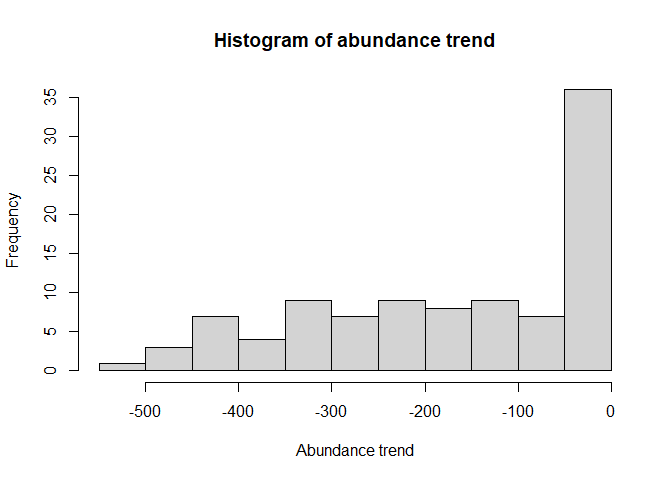hist(metrics_manager$summary_metric_data\$extinction_time,
main = "Histogram of extinction time", xlab = "Extinction time (years BP)")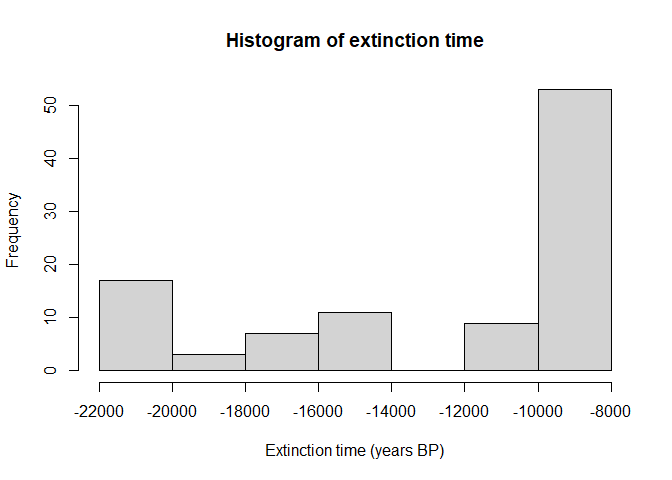Looking at these results, both of these summary metrics, abundance trend over the first ten time steps and extinction time, could be a suitable metric to converge toward a validation target, since there is a spread of outcomes.

From here, you may continue with the poems workflow, as explained in the poems vignette, creating a Validator object for pattern-oriented modeling to validate the results of the simulation.